# MCAT Physical : Newton's Second Law

## Example Questions

← Previous 1

### Example Question #6 : Ap Physics 1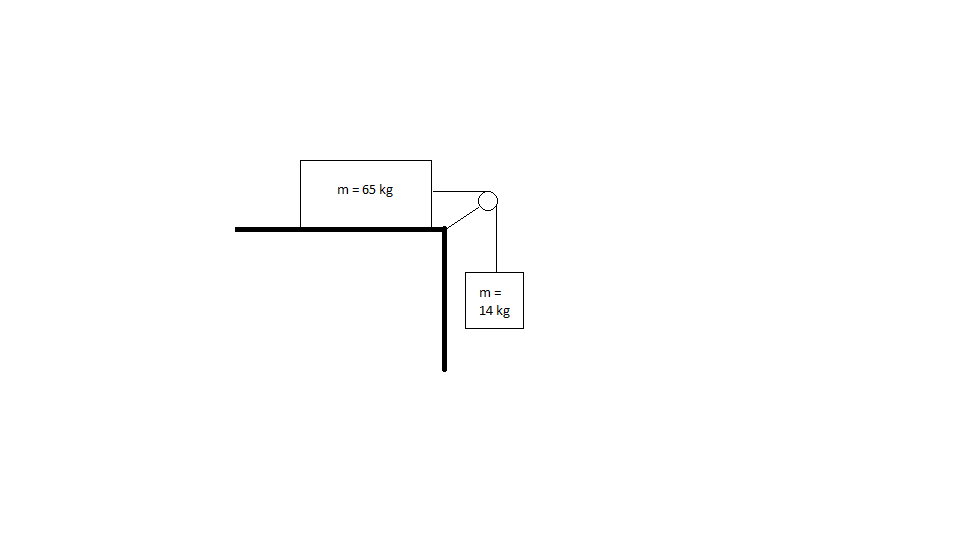What is the acceleration of the system shown above? (Assume the table is frictionless and the mass of the rope connecting blocks is negligible).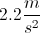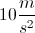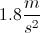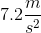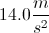Explanation:

The force that translates to the entire system is that of gravity acting on the mass hanging over the ledge.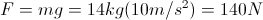140N is the total force acting on the system, which has a mass equal to both blocks combined (65 kg + 14 kg = 79 kg). We can find the acceleration using Newton's second law.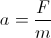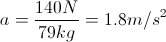### Example Question #1 : Newton's Second Law

A 2kg lead ball is loaded into a spring cannon and the cannon is set at a 45º angle on a platform. The spring has a spring constant of 100N/m and the ball and spring system is compressed by 1m before launch. While the ball is in flight air resistance can be neglected, and the ball finishes its flight by landing at a cushion placed some distance away from the cannon.

What is the acceleration of the ball during launch?

53m/s2

19m/s2

10m/s2

50m/s2

50m/s2

Explanation:

Newton’s second law can be used in combination with the spring force equation to solve for the acceleration of the system. Remember that any force, whether it is kinematic, electrical, or gravitational, is equal to Newton’s second law.

Fspring = kx = masystem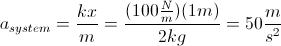### Example Question #2 : Newton's Second Law

A potted plant is hanging from a rope attached to a hook. The plant exerts 25N of force. Assume the rope is weightless.

If the packaging says the rope is able to withstand 800N of force, what is the maximum amount of mass that can be hanging from the rope?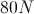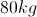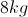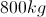Explanation:

If the rope can only withstand 800N of force, we can solve for the mass of the object which would result in this maximum force when hanging from the rope.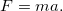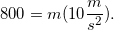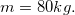### Example Question #3 : Newton's Second Law

A skydiver with a mass of 100kg jumps out of an airplane and reaches a terminal velocity of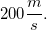.

Which of the following factors will not decrease the total force on the man as he jumps from the plane?

The man jumps as the plane is descending sharply

The man immediately opens his parachute

The man orients his stomach toward the ground, rather than his feet

Increasing the man's mass

Increasing the man's mass

Explanation:

The equation for force isThis shows that by increasing the man's mass, his force will increase after jumping from the plane.

All other options will result in a decreased acceleration when falling. Falling with his stomach downward increases the surface area of the diver, which increases his air resistance. A parachute will also increase the air resistance and surface area. If the plane is descending when he jumps, his time to reach terminal velocity will be reduced, as he will already have an initial velocity in the downward direction.

### Example Question #4 : Newton's Second Law

If a jet accelerates on a runway at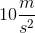and the net force in the horizontal plane is equal to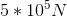, what is the mass of the jet?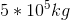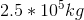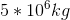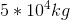Explanation:

To solve this problem use Newton's second law: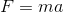We are given the acceleration and the force, both in the horizontal direction. Using these values we can find the mass of the jet.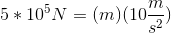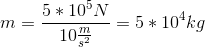### Example Question #5 : Newton's Second Law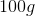mass is suspended by a string, which is passed over a wheel and attached to a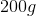mass suspended on the opposite side of the pulley. If the blocks are released, what is the acceleration of the system?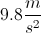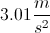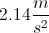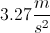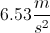Explanation:

An elevator car with its counterweight is a crude example of an Atwood machine, the device described in this question. The acceleration in an Atwood machine varies between zero (the masses are equal) and the full value of gravitational acceleration (one mass is very near zero and the other is very large).

Here, the total mass being accelerated is the sum of the two, or 0.3 kg, so the trick is to find the net force acting on the masses. Although the formal presentation in physics texts looks daunting, just consider that one mass is attempting to fall by pulling up on the other mass, and vice versa. Each experiences a downward force due to gravity: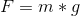This force is transmitted through the string to an upward force on the opposite mass. The net force is simply the gravitational force acting on one mass minus that acting on the other: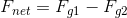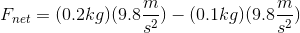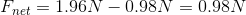Now that we know the net force and the total mass, we can determine the acceleration using Newton's second law: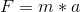.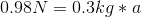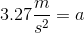You can easily substitute in numbers to see why the Atwood machine has possible solutions betweenand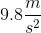. If both masses were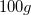, obviously the net force would be zero, and so would the acceleration. If one mass was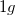and the other was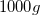, the acceleration would be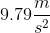, almost equal to gravitational acceleration.

### Example Question #6 : Newton's Second Lawblock lies upon a frictionless surface. A string is attached to the right side of the block, passed over a pulley, and then attached to amass suspended by the string. What is the acceleration of the system when the masses are released?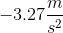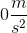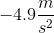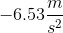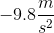Explanation:

The force in the string is all generated by the suspendedmass. This value will be equal to the gravitational force acting on the suspended mass.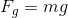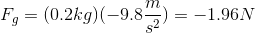Using this force, we can determine the acceleration on the total mass of the system. The total mass being accelerated is: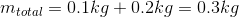Use Newton's second law to calculate the acceleration of the system.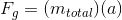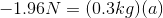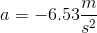Note that the net force and acceleration are negative, indicating that the net movement will be in the downward direction.

It is important to understand that even if the block cannot provide a frictional force to resist being pulled to the right, it still has to be accelerated; Newton's second law is obeyed in frictionless and gravity-free situations (like outer space). This issue is well demonstrated with an Atwood machine.

### Example Question #2 : Ap Physics 1

If a 15kg object is subjected to a force of 175N to the east and another force of 1020N to the west, what is the object’s acceleration?

5.6m/s2

56m/s2

1.4m/s2

113m/s2

56m/s2

Explanation:

First, find the net force by subtracting the opposing forces.

F = 1020N – 175N = 845 N to the west

Next, find acceleration using Newton's second law,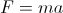.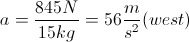### Example Question #7 : Newton's Second Law

A basketball is thrown in the air with an initial velocity of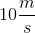, at an angle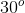above the horizontal. At what point is the ball's acceleration the greatest?

Just after being thrown

Just before the ball lands

The acceleration is constant

At the highest point in the ball's path

The acceleration cannot be determined without know the force on the ball

The acceleration is constant

Explanation:

The only force acting on the ball at any point during flight is the downwards force of gravity, which is constant. There are no forces acting on the ball in the horizontal direction. Any acceleration will result in a force, according to Newton's second law.If there are no other forces, then we can assume that there are no other accelerations acting on the ball. Acceleration due to gravity is constant. If this is the only acceleration on the ball, then the acceleration must be constant throughout flight.

### Example Question #1 : Understanding Gravity And Gravitational Forces

What is the acceleration due to gravity on a planet on which an object with a mass of 20.0kg has a weight of 270N?

27m/s2

13.5m/s2

0.074m/s2

10m/s 2

13.5m/s2

Explanation:

Solve the following equation for acceleration, using the values given in the question.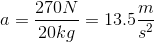← Previous 1